# The Magnetic Pendulum

This article is based on an article named "Experimente zum Chaos" (translated.: "Experiments on Chaos")  from the german magazine Spektrum der Wissenschaft. The original article introduces, among other things a simulation showing the chaotic motion of a pendulum under the influence of gravity and three magnets. This article will provide source code and explanations of the science behind simulating such a magnetic pendulum.The magnetic pendulum fractal: A pattern created by a run of the magnetic pendulum simulation. Three magnets are distributed along the edges of an equilateral triangle. The pendulum mount point is in the center of the image.

The simulation is based on computing the motion of a magnetic pendulum under the influence of three magnets. A color image is obtained by integrating the equations of motion of all possible starting points in a two dimensional grid and coloring the starting point with the color of the magnet over which the pendulum came to a rest.

When running the simulation you will see that a minor variation in the starting point of the pendulum will result in a vastly different pendulum motion after some time. Scientifically spoken one can say that the result of the simulation is highly sensitive to small variations in its initial conditions. The effect that small variations in the initial conditions of a dynamical system will result in large, seemingly unpredictable variations of the result is investigated by a special branch of mathematics, the so called "Chaos Theory".

In 1963, Edward Lorenz an american mathematician and meteorologist discovered a similar behavior when studying the influence of initial conditions on simulations used for weather predictions. He wrote:

One meteorologist remarked that if the theory were correct, one flap of a sea gull's wings would be enough to alter the course of the weather forever. The controversy has not yet been settled, but the most recent evidence seems to favor the sea gulls. 

In later speeches the "seagull" was transformed into a more poetic "butterfly" and the term "Butterfly effect" was born to describe the phenomenon.

The source code of the pendulum simulation embedded here is written in Typescript. It is part of the Educational-Javascripts-Typescript archive and can be downloaded from GitHub.

## The Simulation Model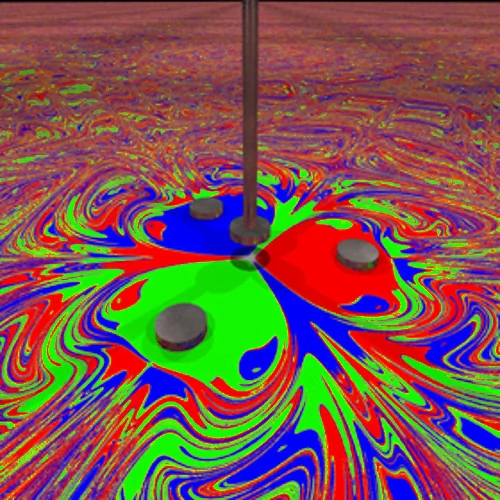Rendering of the experimental setup (image courtesy of Paul Nylander).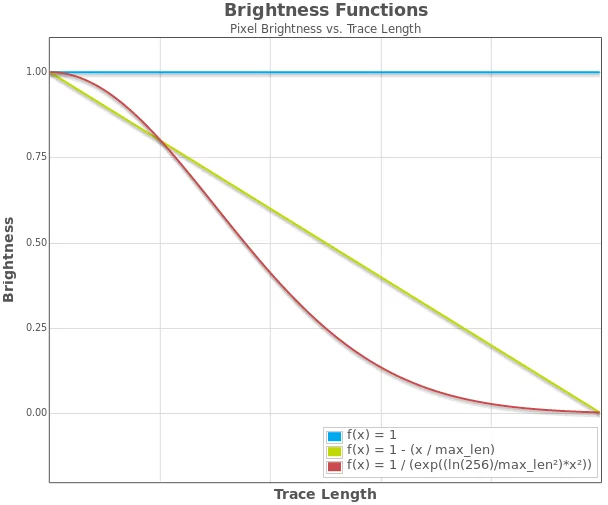Overview over different functions for computing pixel brightness and the effect of using them.

In this section I will describe the underlying physical model. In the end you will see that small changes in the initial conditions and the environment of the simulation can result in large variations of the result. This will ultimately lead to a certain level of unpredictability in the simulated pendulum endpoint.

The model assumes that we are dealing with a magnetic pendulum that is attracted by three magnets. Each of the magnets is assigned a distinct color (red, green or blue). The magnets are located on the edges of a equilateral triangle with the pendulum mount point being at the center. The magnetic force is strong enough to attract the pendulum in a way that it will not come to rest in the center position. The image to the right shows a rendering of the model setup.

The simulation will calculate the route taken by the pendulum under the influence of all three magnets as well as gravity and friction. Due to energy loss caused by friction, the pendulum will stop earlier or later over one of the magnets. The starting point is then colored with the color of this magnet. Doing this for all pixels will result in a map showing a pattern composed of red, green, and blue pixels. The applet above can be used to show sample traces. The friction can be adjusted with a slider.

For obtaining better looking results it is advisable to vary the brightness of each pixel. This can be done by taking the length of the pendulum trace into account. The diagram to the right shows three different color functions. Those functions take the length of the pendulum trace as their only parameter. Starting points resulting in longer traces are shown in darker colors thus adding additional depth to the image.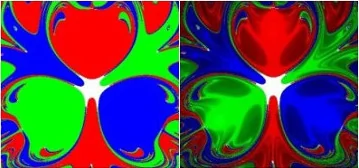The effect of darkening colors with increasing trace length

### Simplifications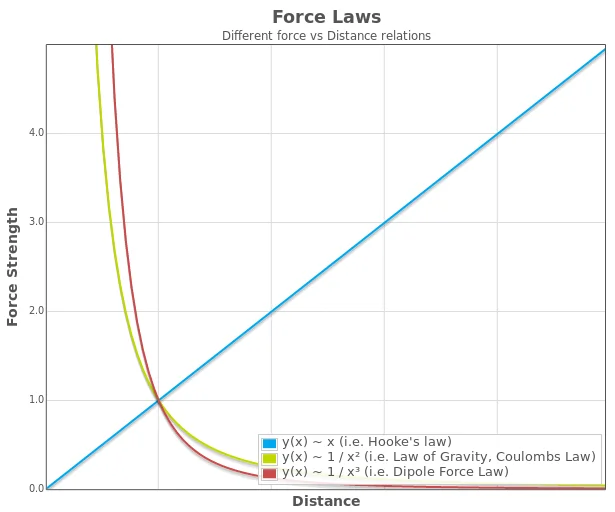Example curves of Force vs. Distance for Hooke's and Magnetic Forces.

The pendulum is a simplified 2D version. The assumption is that the force pulling it back to the centre is proportional to the distance (Hookes law). This is a simplification to avoid computing the exact physics on the surface of a sphere. The simplification is justified if the pendulum is long.

Magnets are assumed to cause a force proportional to the inverse square of the pendulum distance. This is akin to the Law of gravity or Coloumbs law. In reality the magnetic force is a dipol force which is proportional to 1/r³ rather than 1/r². You can change the force law in the configuration file of the application provided with this article.

### Equations of motion

The pendulum movement is calculated by integrating twice over the accelerations acting on the pendulum. Normally, one would not talk about accelerations but forces. According to Newton's second law of motion, the force necessary to move a body equals mass times acceleration. We solve that equation for the acceleration:

\begin{equation} \nonumber\vec{F} = m \vec{a} \end{equation} \begin{equation} \nonumber\vec{a} = {\vec{F} \over m} \end{equation}

The initial conditions will provide a starting position and a starting velocity. From there all we need to do is compute the accelerations and apply Newtons second law. For simplicity, mass is assumed to equal one mass unit. The following equations list all accelerations relevant for the simulation:

Acceleration caused by the pullback of the pendulum:

\begin{equation} \nonumber\vec{a}_g = k_g \cdot \vec{r} \end{equation}

Accelleration caused by a single magnet:

\begin{equation} \nonumber\vec{a}_m = k_m \cdot \frac{\vec{r}}{|\vec{r}|^3} \end{equation}

Decelleration caused by friction:

\begin{equation} \nonumber \vec{a}_f = -k_f \cdot \vec{v} \end{equation}

Total acceleration of the pendulum:

\begin{equation} \nonumber \vec{a}_t = \vec{a}_g + \Big( \sum_{m=1}^3{\vec{a}_m} \Big) - \vec{a}_f \end{equation}

with:

• $$\vec{a}_t$$ - Total acceleration of the pendulum
• $$\vec{a}_g$$ - Acceleration caused by the pullback of the pendulum
• $$\vec{a}_m$$ - Acceleration caused by the magnet m
• $$\vec{a}_f$$ - Deceleration caused by friction
• $$k_f$$ - Coefficient of friction
• $$k_g$$ - Strength of the pendulum pullback
• $$k_m$$ - Strength of the magnet
• $$\vec{r}$$ - Position vector of the pendulum

### Integration of the Pendulum trace

Given an initial pendulum position and an initial pendulum velocity, all that is left to do is find a suitable integration scheme and follow the pendulum's trail. For this simulation, the Beeman integration algorithm was used. Applying this scheme does not require much code, and it is pretty accurate.

for (int ct=0; ct<m_nMaxSteps && bRunning; ++ct)
{
// compute new position
pos += vel*dt + sqr(dt) * (2.0/3.0 * (*acc) - 1.0 / 6.0 * (*acc_p));
pos += vel*dt + sqr(dt) * (2.0/3.0 * (*acc) - 1.0 / 6.0 * (*acc_p));

(*acc_n) = 0.0;    // reset accelleration

// Calculate Force, we deal with Forces proportional
// to the distance or the inverse square of the distance
for (std::size_t i=0; i<src_num; ++i)
{
const Source &src( m_vSources[i] );
r = pos - src.pos;
if (src.type==Source::EType::tpLIN)
{
// Hooke's law:          _
//         _             r         _
//  m * a = - k * |r| * --- = -k * r
//                      |r|
//
(*acc_n) -= src.mult * r;
(*acc_n) -= src.mult * r;
}
else
{
// Magnet Forces: _
//      _         r
//  m * a = k * -----
//               |r³|
//
double dist( sqrt( sqr(src.pos - pos) +
sqr(src.pos - pos) +
sqr(m_fHeight) ) );
(*acc_n) -= (src.mult / (dist*dist*dist)) * r;
(*acc_n) -= (src.mult / (dist*dist*dist)) * r;
}

// Check abort condition
if (ct>m_nMinSteps && abs(r)<src.size && abs(vel)<m_fAbortVel)
{
bRunning = false;
stop_mag = (int)i;
break;
}
}   // for all sources

// 3.) Friction proportional to velocity
(*acc_n) -= vel * m_fFriction;
(*acc_n) -= vel * m_fFriction;

// 4.) Beeman integration for velocities
vel += dt * ( 1.0/3.0 * (*acc_n) + 5.0/6.0 * (*acc) - 1.0/6.0 * (*acc_p) );
vel += dt * ( 1.0/3.0 * (*acc_n) + 5.0/6.0 * (*acc) - 1.0/6.0 * (*acc_p) );

// 5.) flip the acc buffer
tmp = acc_p;
acc_p = acc;
acc   = acc_n;
acc_n = tmp;
}

The archive requires Windows and the Visual Studio development environment. A precompiled executable is contained in the archive. The executable can also be used under Linux with wine.

A documentation of the C++ sample application can be found here

## Gallery

The images and animations displayed here were computed with the C++ application.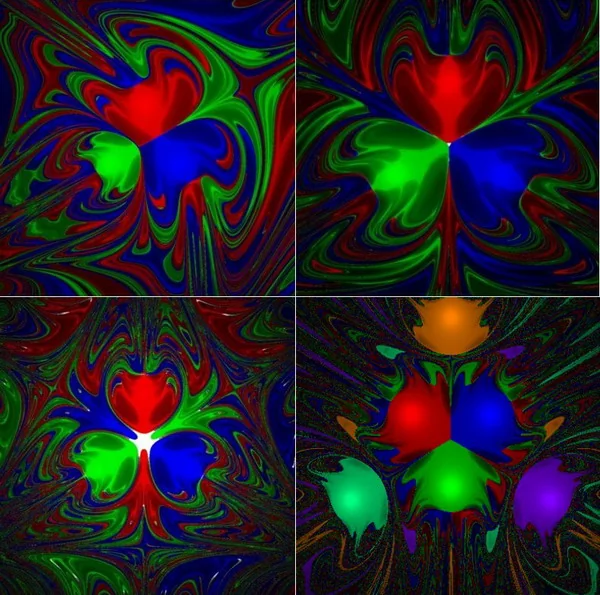A selection of images computed with different parameters.
An animation showing color patterns for a rotating magnet configuration.

## References

1. “Experimente zum Chaos.” Sascha Hilgenfeldt and HANS-Christoph Schulz, Specktrum der Wissenschaft 1, 1994, Seite 72
2. “Butterfly Effect.” Wikipedia: The Free Encyclopedia. Wikimedia Foundation, Inc., Web. Date accessed (01 February 2015).
3. “Fractals” A webpage by Paul Nylander, Web.
4. "The Predictability of Hydrodynamic Flow" Lorenz, Edward N. (1963). Transactions of the New York Academy of Sciences. 25 (4): 409–432.